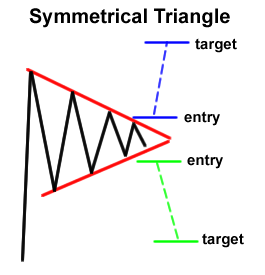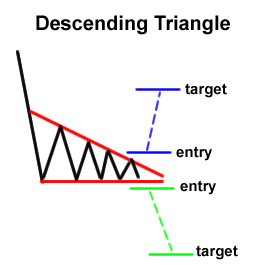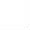Browse Courses# Ins & Outs of Triangle Patterns

Jul 2015

Triangles are believed to be the most essential and frequently emerged chart patterns in forex trading. They are sometimes termed as the holy grail of day trading because of their reliability and effectiveness. In this article we are going to discuss the main types of triangle patterns and how to trade them.

## Types of Triangle Patterns

Triangles are of mainly three types that include;

• Symmetrical triangle
• Ascending Triangle
• Descending Triangle

Each type is further divided into bullish and bearish categories depending on the direction of the breakout. Let’s discuss each one by one.

## Symmetrical Triangle

In this triangle both the trendlines are symmetrical or parallel to each other as obvious by its name. Symmetrical triangle shows consolidation phase which is followed by a major breakout in any direction.

### How to Trade Symmetrical Triangle Breakout?

The Symmetrical Triangle Breakout may be traded in two ways depending on the direction of the breakout as demonstrated in the following diagram;A Buy Stop or Instant Buy order may be placed if the price breaks the upper trendline. The target may be in accordance with your money management rules. Generally a risk/reward ratio of at least 1.5 is considered good.

On the other hand, a Sell Stop or Instant Sell order should be placed if the price breaks the lower trendline. The Symmetrical Triangle Breakout happens once a candle is closed below or above the trendline. The reliability of triangles depends on the timeframe i.e. higher the timeframe, more reliable the triangle will be.

There are two types of Symmetrical Triangle Formation: Bullish Symmetrical Triangle and Bearish Symmetrical Triangle. If the price breaks the upper trendline then such a formation is known as Bullish Symmetrical Triangle while in case of lower trendline breakout, the triangle is called Bearish Symmetrical Triangle.

## Ascending Triangle

Ascending triangle formation is the one with upward direction due to rising lower trendline. Ascending triangle chart pattern shows upward consolidation phase which usually follows a strong bullish momentum.

### How to Trade Ascending Triangle Breakout?

Ascending triangle formation can be of two types. If the price breaks the upper trendline then such a formation is called Bullish Ascending Triangle formation while if the price breaks the lower trendline then the formation is known as Bearish Ascending Triangle formation.

In order to trade bullish ascending triangle formation, a Buy Stop or Instant Buy order may be placed just above the upper trendline while in case of bearish ascending triangle, a Sell Stop or Instant Sell order should be placed as demonstrated in the following diagram.Research shows that in more than 60% cases, ascending triangle ends with a bullish breakout. Proper risk/reward ratio is always recommended while trading ascending triangles.

## Descending Triangle

A triangle with falling upper trendline and straight lower trendline is known as Descending Triangle Formation. Descending triangle shows the consolidation phase after a major downside move.

### How to Trade Descending Triangle Breakout

Just as the above mentioned two triangles, the descending triangle is also of two types. If the price breaks the upper trendline then such as triangle is known as bullish descending triangle while in case of downside breakout the triangle is called bearish descending triangle.Descending triangles are traded in the same way as we discussed in case of Symmetrical and Ascending triangles. Research shows that over 70% of descending triangles end with a bearish breakout.

## Conclusion

Triangle patterns are frequently appeared formations in forex trading that may help optimize profitability with minimal risk if traded correctly.